# Mean State

Period Mean (original grids) [Watt m-2]
Model Period Mean (intersection) [Watt m-2]
Model Period Mean (complement) [Watt m-2]
Benchmark Period Mean (intersection) [Watt m-2]
Benchmark Period Mean (complement) [Watt m-2]
Bias [Watt m-2]
RMSE [Watt m-2]
Phase Shift [months]
Bias Score 
RMSE Score 
Seasonal Cycle Score 
Spatial Distribution Score 
Interannual Variability Score 
Overall Score 
Benchmark [-] 122.
CRUNCEPv7 [-] 135. 135. 0.00 120. 164. 15.3 25.4 1.44 0.39 0.41 0.80 0.97 0.55 0.59
GSWP3v1 [-] 116. 116. 0.00 120. 164. -3.73 17.7 0.987 0.68 0.44 0.87 0.89 0.67 0.67
WATCH [-] 86.6 86.4 0.00 122. 165. -34.6 41.0 2.19 0.22 0.36 0.65 0.82 0.72 0.52
Period Mean (original grids) [Watt m-2]
Model Period Mean (intersection) [Watt m-2]
Model Period Mean (complement) [Watt m-2]
Benchmark Period Mean (intersection) [Watt m-2]
Benchmark Period Mean (complement) [Watt m-2]
Bias [Watt m-2]
RMSE [Watt m-2]
Phase Shift [months]
Bias Score 
RMSE Score 
Seasonal Cycle Score 
Spatial Distribution Score 
Interannual Variability Score 
Overall Score 
Benchmark [-] 102.
CRUNCEPv7 [-] 108. 107. 0.00 101. 165. 6.68 23.4 1.50 0.65 0.46 0.78 0.99 0.62 0.66
GSWP3v1 [-] 97.7 97.2 0.00 101. 165. -3.30 19.8 1.28 0.67 0.51 0.81 0.89 0.65 0.67
WATCH [-] 78.7 78.6 0.00 103. 166. -23.3 33.6 1.61 0.43 0.44 0.76 0.72 0.65 0.57
Period Mean (original grids) [Watt m-2]
Model Period Mean (intersection) [Watt m-2]
Model Period Mean (complement) [Watt m-2]
Benchmark Period Mean (intersection) [Watt m-2]
Benchmark Period Mean (complement) [Watt m-2]
Bias [Watt m-2]
RMSE [Watt m-2]
Phase Shift [months]
Bias Score 
RMSE Score 
Seasonal Cycle Score 
Spatial Distribution Score 
Interannual Variability Score 
Overall Score 
Benchmark [-] 112.
CRUNCEPv7 [-] 113. 113. 0.00 110. 159. 5.35 22.1 1.18 0.67 0.56 0.84 0.96 0.55 0.69
GSWP3v1 [-] 105. 105. 0.00 110. 159. -3.73 20.8 1.22 0.71 0.57 0.81 0.87 0.61 0.69
WATCH [-] 99.8 99.7 0.00 112. 159. -11.5 27.2 2.18 0.54 0.51 0.66 0.57 0.66 0.57
Period Mean (original grids) [Watt m-2]
Model Period Mean (intersection) [Watt m-2]
Model Period Mean (complement) [Watt m-2]
Benchmark Period Mean (intersection) [Watt m-2]
Benchmark Period Mean (complement) [Watt m-2]
Bias [Watt m-2]
RMSE [Watt m-2]
Phase Shift [months]
Bias Score 
RMSE Score 
Seasonal Cycle Score 
Spatial Distribution Score 
Interannual Variability Score 
Overall Score 
Benchmark [-] 43.3
CRUNCEPv7 [-] 14.6 14.7 0.00 43.2 44.3 -29.9 33.5 0.368 0.59 0.70 0.98 0.76 0.64 0.73
GSWP3v1 [-] 33.3 33.3 0.00 43.2 44.3 -11.6 23.4 0.172 0.81 0.70 0.99 0.78 0.76 0.79
WATCH [-] 22.1 22.2 0.00 43.8 45.2 -21.5 26.6 0.200 0.68 0.73 0.99 0.97 0.76 0.81
Period Mean (original grids) [Watt m-2]
Model Period Mean (intersection) [Watt m-2]
Model Period Mean (complement) [Watt m-2]
Benchmark Period Mean (intersection) [Watt m-2]
Benchmark Period Mean (complement) [Watt m-2]
Bias [Watt m-2]
RMSE [Watt m-2]
Phase Shift [months]
Bias Score 
RMSE Score 
Seasonal Cycle Score 
Spatial Distribution Score 
Interannual Variability Score 
Overall Score 
Benchmark [-] 144.
CRUNCEPv7 [-] 157. 156. 0.00 140. 160. 20.0 30.5 1.51 0.35 0.39 0.78 0.84 0.48 0.54
GSWP3v1 [-] 128. 127. 0.00 140. 160. -7.88 20.6 0.968 0.60 0.43 0.86 0.90 0.60 0.64
WATCH [-] 103. 103. 0.00 141. 161. -31.1 37.6 1.68 0.26 0.36 0.74 0.92 0.73 0.56
Period Mean (original grids) [Watt m-2]
Model Period Mean (intersection) [Watt m-2]
Model Period Mean (complement) [Watt m-2]
Benchmark Period Mean (intersection) [Watt m-2]
Benchmark Period Mean (complement) [Watt m-2]
Bias [Watt m-2]
RMSE [Watt m-2]
Phase Shift [months]
Bias Score 
RMSE Score 
Seasonal Cycle Score 
Spatial Distribution Score 
Interannual Variability Score 
Overall Score 
Benchmark [-] 62.5
CRUNCEPv7 [-] 42.4 42.4 0.00 62.3 75.7 -19.8 31.9 0.344 0.71 0.65 0.98 0.88 0.66 0.75
GSWP3v1 [-] 48.1 48.1 0.00 62.3 75.7 -13.9 23.3 0.107 0.79 0.72 0.99 0.94 0.67 0.80
WATCH [-] 39.0 38.9 0.00 63.6 76.5 -24.3 29.9 0.0958 0.65 0.74 0.99 0.99 0.69 0.80
Period Mean (original grids) [Watt m-2]
Model Period Mean (intersection) [Watt m-2]
Model Period Mean (complement) [Watt m-2]
Benchmark Period Mean (intersection) [Watt m-2]
Benchmark Period Mean (complement) [Watt m-2]
Bias [Watt m-2]
RMSE [Watt m-2]
Phase Shift [months]
Bias Score 
RMSE Score 
Seasonal Cycle Score 
Spatial Distribution Score 
Interannual Variability Score 
Overall Score 
Benchmark [-] 72.7
CRUNCEPv7 [-] 66.3 66.0 0.00 72.1 94.9 -4.76 30.0 0.732 0.71 0.57 0.93 0.89 0.64 0.72
GSWP3v1 [-] 62.7 62.6 0.00 72.1 94.9 -8.19 21.7 0.393 0.79 0.65 0.97 0.99 0.63 0.78
WATCH [-] 58.4 58.1 0.00 74.8 95.6 -15.6 27.1 0.573 0.69 0.64 0.95 0.98 0.67 0.76
Period Mean (original grids) [Watt m-2]
Model Period Mean (intersection) [Watt m-2]
Model Period Mean (complement) [Watt m-2]
Benchmark Period Mean (intersection) [Watt m-2]
Benchmark Period Mean (complement) [Watt m-2]
Bias [Watt m-2]
RMSE [Watt m-2]
Phase Shift [months]
Bias Score 
RMSE Score 
Seasonal Cycle Score 
Spatial Distribution Score 
Interannual Variability Score 
Overall Score 
Benchmark [-] 109.
CRUNCEPv7 [-] 121. 121. 0.00 105. 157. 20.0 33.3 0.825 0.46 0.50 0.92 0.99 0.52 0.65
GSWP3v1 [-] 94.4 94.1 0.00 105. 157. -6.92 23.7 0.544 0.72 0.53 0.95 0.96 0.56 0.71
WATCH [-] 83.6 83.8 0.00 108. 157. -20.2 33.6 0.818 0.52 0.48 0.92 0.92 0.59 0.65
Period Mean (original grids) [Watt m-2]
Model Period Mean (intersection) [Watt m-2]
Model Period Mean (complement) [Watt m-2]
Benchmark Period Mean (intersection) [Watt m-2]
Benchmark Period Mean (complement) [Watt m-2]
Bias [Watt m-2]
RMSE [Watt m-2]
Phase Shift [months]
Bias Score 
RMSE Score 
Seasonal Cycle Score 
Spatial Distribution Score 
Interannual Variability Score 
Overall Score 
Benchmark [-] 41.8
CRUNCEPv7 [-] 9.84 10.2 0.00 41.9 41.5 -32.9 33.8 0.382 0.54 0.67 0.97 0.71 0.70 0.71
GSWP3v1 [-] 25.1 25.4 0.00 41.9 41.5 -17.5 23.3 0.253 0.72 0.70 0.98 0.94 0.75 0.80
WATCH [-] 15.8 16.0 0.00 42.3 42.2 -26.6 28.4 0.257 0.61 0.70 0.98 0.95 0.73 0.78
Period Mean (original grids) [Watt m-2]
Model Period Mean (intersection) [Watt m-2]
Model Period Mean (complement) [Watt m-2]
Benchmark Period Mean (intersection) [Watt m-2]
Benchmark Period Mean (complement) [Watt m-2]
Bias [Watt m-2]
RMSE [Watt m-2]
Phase Shift [months]
Bias Score 
RMSE Score 
Seasonal Cycle Score 
Spatial Distribution Score 
Interannual Variability Score 
Overall Score 
Benchmark [-] 96.5
CRUNCEPv7 [-] 97.5 97.5 0.00 93.2 138. 5.79 23.2 0.580 0.72 0.64 0.95 0.93 0.60 0.75
GSWP3v1 [-] 92.5 92.4 0.00 93.2 138. 1.15 20.2 0.330 0.78 0.67 0.97 0.87 0.64 0.76
WATCH [-] 101. 101. 0.00 96.0 139. 5.68 24.4 0.645 0.70 0.63 0.95 0.73 0.71 0.73
Period Mean (original grids) [Watt m-2]
Model Period Mean (intersection) [Watt m-2]
Model Period Mean (complement) [Watt m-2]
Benchmark Period Mean (intersection) [Watt m-2]
Benchmark Period Mean (complement) [Watt m-2]
Bias [Watt m-2]
RMSE [Watt m-2]
Phase Shift [months]
Bias Score 
RMSE Score 
Seasonal Cycle Score 
Spatial Distribution Score 
Interannual Variability Score 
Overall Score 
Benchmark [-] 64.4
CRUNCEPv7 [-] 60.9 61.0 0.00 61.9 90.5 -0.150 25.5 0.326 0.81 0.67 0.98 0.88 0.74 0.79
GSWP3v1 [-] 54.4 54.4 0.00 61.9 90.5 -5.49 19.0 0.289 0.86 0.74 0.98 0.98 0.64 0.82
WATCH [-] 40.5 40.5 0.00 62.1 90.4 -19.5 25.6 0.329 0.72 0.76 0.98 0.97 0.67 0.81
Period Mean (original grids) [Watt m-2]
Model Period Mean (intersection) [Watt m-2]
Model Period Mean (complement) [Watt m-2]
Benchmark Period Mean (intersection) [Watt m-2]
Benchmark Period Mean (complement) [Watt m-2]
Bias [Watt m-2]
RMSE [Watt m-2]
Phase Shift [months]
Bias Score 
RMSE Score 
Seasonal Cycle Score 
Spatial Distribution Score 
Interannual Variability Score 
Overall Score 
Benchmark [-] 115.
CRUNCEPv7 [-] 71.5 71.5 0.00 77.4 133. -4.18 29.2 0.799 0.59 0.56 0.91 0.86 0.56 0.68
GSWP3v1 [-] 65.7 65.7 0.00 77.4 133. -10.1 23.7 0.563 0.68 0.60 0.93 0.92 0.62 0.73
WATCH [-] 58.8 58.8 0.00 78.9 133. -18.6 29.6 0.863 0.57 0.58 0.89 0.93 0.65 0.70
Period Mean (original grids) [Watt m-2]
Model Period Mean (intersection) [Watt m-2]
Model Period Mean (complement) [Watt m-2]
Benchmark Period Mean (intersection) [Watt m-2]
Benchmark Period Mean (complement) [Watt m-2]
Bias [Watt m-2]
RMSE [Watt m-2]
Phase Shift [months]
Bias Score 
RMSE Score 
Seasonal Cycle Score 
Spatial Distribution Score 
Interannual Variability Score 
Overall Score 
Benchmark [-] 54.9
CRUNCEPv7 [-] 64.5 64.6 0.00 51.3 143. 15.6 32.9 1.47 0.52 0.47 0.80 0.88 0.50 0.61
GSWP3v1 [-] 54.2 54.2 0.00 51.3 143. 5.15 26.1 0.880 0.66 0.52 0.89 0.85 0.55 0.67
WATCH [-] 43.5 43.6 0.00 54.6 144. -9.13 26.8 1.28 0.67 0.51 0.83 0.80 0.54 0.64
Period Mean (original grids) [Watt m-2]
Model Period Mean (intersection) [Watt m-2]
Model Period Mean (complement) [Watt m-2]
Benchmark Period Mean (intersection) [Watt m-2]
Benchmark Period Mean (complement) [Watt m-2]
Bias [Watt m-2]
RMSE [Watt m-2]
Phase Shift [months]
Bias Score 
RMSE Score 
Seasonal Cycle Score 
Spatial Distribution Score 
Interannual Variability Score 
Overall Score 
Benchmark [-] 44.9
CRUNCEPv7 [-] 15.2 15.0 0.00 44.8 45.6 -31.6 35.1 0.181 0.57 0.70 0.99 0.75 0.64 0.72
GSWP3v1 [-] 28.4 28.3 0.00 44.8 45.6 -18.6 24.6 0.122 0.72 0.72 0.99 0.87 0.78 0.80
WATCH [-] 22.8 22.6 0.00 45.3 46.2 -23.3 27.7 0.135 0.66 0.72 0.99 0.92 0.78 0.80
Period Mean (original grids) [Watt m-2]
Model Period Mean (intersection) [Watt m-2]
Model Period Mean (complement) [Watt m-2]
Benchmark Period Mean (intersection) [Watt m-2]
Benchmark Period Mean (complement) [Watt m-2]
Bias [Watt m-2]
RMSE [Watt m-2]
Phase Shift [months]
Bias Score 
RMSE Score 
Seasonal Cycle Score 
Spatial Distribution Score 
Interannual Variability Score 
Overall Score 
Benchmark [-] 134.
CRUNCEPv7 [-] 116. 116. 0.00 127. 169. -2.92 27.2 1.04 0.57 0.59 0.88 0.96 0.57 0.69
GSWP3v1 [-] 107. 108. 0.00 127. 169. -14.0 24.1 0.799 0.64 0.60 0.90 0.95 0.62 0.72
WATCH [-] 99.8 99.9 0.00 127. 169. -22.2 32.1 1.16 0.51 0.56 0.85 0.70 0.66 0.64
Period Mean (original grids) [Watt m-2]
Model Period Mean (intersection) [Watt m-2]
Model Period Mean (complement) [Watt m-2]
Benchmark Period Mean (intersection) [Watt m-2]
Benchmark Period Mean (complement) [Watt m-2]
Bias [Watt m-2]
RMSE [Watt m-2]
Phase Shift [months]
Bias Score 
RMSE Score 
Seasonal Cycle Score 
Spatial Distribution Score 
Interannual Variability Score 
Overall Score 
Benchmark [-] 99.6
CRUNCEPv7 [-] 89.4 89.7 0.00 97.8 136. -6.20 25.6 0.491 0.81 0.63 0.96 0.81 0.58 0.74
GSWP3v1 [-] 82.0 82.1 0.00 97.8 136. -14.0 21.5 0.352 0.75 0.72 0.97 1.0 0.62 0.80
WATCH [-] 82.8 82.9 0.00 96.7 135. -12.6 22.1 0.406 0.78 0.70 0.97 0.99 0.68 0.80
Period Mean (original grids) [Watt m-2]
Model Period Mean (intersection) [Watt m-2]
Model Period Mean (complement) [Watt m-2]
Benchmark Period Mean (intersection) [Watt m-2]
Benchmark Period Mean (complement) [Watt m-2]
Bias [Watt m-2]
RMSE [Watt m-2]
Phase Shift [months]
Bias Score 
RMSE Score 
Seasonal Cycle Score 
Spatial Distribution Score 
Interannual Variability Score 
Overall Score 
Benchmark [-] 72.2
CRUNCEPv7 [-] 54.7 54.9 0.00 72.0 77.2 -17.2 29.2 0.370 0.74 0.66 0.97 0.96 0.61 0.77
GSWP3v1 [-] 58.6 58.7 0.00 72.0 77.2 -13.2 20.6 0.102 0.79 0.76 0.99 1.0 0.67 0.83
WATCH [-] 54.9 55.1 0.00 72.3 76.9 -16.8 24.0 0.218 0.74 0.75 0.99 0.97 0.69 0.81
Period Mean (original grids) [Watt m-2]
Model Period Mean (intersection) [Watt m-2]
Model Period Mean (complement) [Watt m-2]
Benchmark Period Mean (intersection) [Watt m-2]
Benchmark Period Mean (complement) [Watt m-2]
Bias [Watt m-2]
RMSE [Watt m-2]
Phase Shift [months]
Bias Score 
RMSE Score 
Seasonal Cycle Score 
Spatial Distribution Score 
Interannual Variability Score 
Overall Score 
Benchmark [-] 81.6
CRUNCEPv7 [-] 64.3 64.9 0.00 81.1 116. -15.5 26.1 0.532 0.70 0.68 0.96 0.99 0.63 0.77
GSWP3v1 [-] 72.0 72.2 0.00 81.1 116. -8.22 20.4 0.314 0.79 0.71 0.98 0.99 0.66 0.81
WATCH [-] 72.1 72.5 0.00 82.2 115. -8.94 22.7 0.465 0.79 0.68 0.96 0.99 0.72 0.80
Period Mean (original grids) [Watt m-2]
Model Period Mean (intersection) [Watt m-2]
Model Period Mean (complement) [Watt m-2]
Benchmark Period Mean (intersection) [Watt m-2]
Benchmark Period Mean (complement) [Watt m-2]
Bias [Watt m-2]
RMSE [Watt m-2]
Phase Shift [months]
Bias Score 
RMSE Score 
Seasonal Cycle Score 
Spatial Distribution Score 
Interannual Variability Score 
Overall Score 
Benchmark [-] 132.
CRUNCEPv7 [-] 151. 151. 0.00 131. 178. 20.5 27.7 1.16 0.33 0.42 0.84 0.92 0.51 0.57
GSWP3v1 [-] 122. 122. 0.00 131. 178. -7.96 20.3 0.991 0.62 0.39 0.86 0.95 0.60 0.64
WATCH [-] 114. 114. 0.00 129. 177. -14.7 30.6 1.73 0.43 0.34 0.75 0.98 0.67 0.58
Period Mean (original grids) [Watt m-2]
Model Period Mean (intersection) [Watt m-2]
Model Period Mean (complement) [Watt m-2]
Benchmark Period Mean (intersection) [Watt m-2]
Benchmark Period Mean (complement) [Watt m-2]
Bias [Watt m-2]
RMSE [Watt m-2]
Phase Shift [months]
Bias Score 
RMSE Score 
Seasonal Cycle Score 
Spatial Distribution Score 
Interannual Variability Score 
Overall Score 
Benchmark [-] 117.
CRUNCEPv7 [-] 121. 121. 0.00 117. 145. 5.09 21.7 1.01 0.71 0.55 0.88 0.93 0.55 0.69
GSWP3v1 [-] 108. 108. 0.00 117. 145. -8.04 20.3 0.874 0.73 0.56 0.88 0.97 0.62 0.72
WATCH [-] 102. 103. 0.00 116. 144. -13.2 25.3 1.41 0.62 0.53 0.79 0.99 0.67 0.69
Period Mean (original grids) [Watt m-2]
Model Period Mean (intersection) [Watt m-2]
Model Period Mean (complement) [Watt m-2]
Benchmark Period Mean (intersection) [Watt m-2]
Benchmark Period Mean (complement) [Watt m-2]
Bias [Watt m-2]
RMSE [Watt m-2]
Phase Shift [months]
Bias Score 
RMSE Score 
Seasonal Cycle Score 
Spatial Distribution Score 
Interannual Variability Score 
Overall Score 
Benchmark [-] 95.7
CRUNCEPv7 [-] 81.9 81.5 0.00 92.5 124. -10.7 27.6 0.604 0.71 0.66 0.94 0.91 0.52 0.73
GSWP3v1 [-] 90.8 90.1 0.00 92.5 124. -3.40 24.9 0.391 0.74 0.68 0.96 0.99 0.57 0.77
WATCH [-] 87.0 86.6 0.00 92.9 124. -6.76 27.1 0.666 0.73 0.65 0.92 0.96 0.56 0.74

# Temporally integrated period mean

BENCHMARK MEAN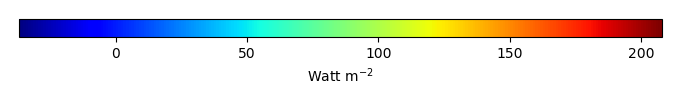MODEL MEANBIAS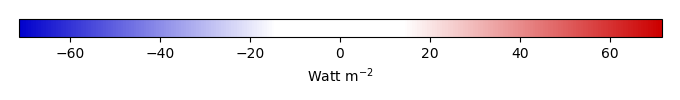BIAS SCORERMSE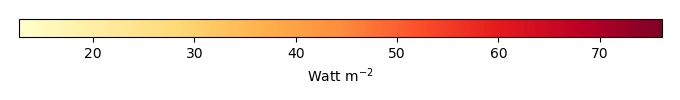RMSE SCOREBENCHMARK INTERANNUAL VARIABILITY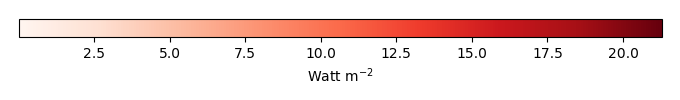MODEL INTERANNUAL VARIABILITYINTERANNUAL VARIABILITY SCOREBENCHMARK MAX MONTHMODEL MAX MONTHDIFFERENCE IN MAX MONTH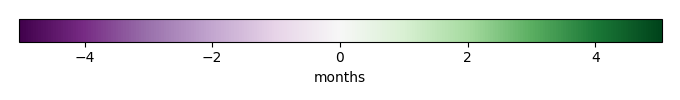SEASONAL CYCLE SCORESPATIAL TAYLOR DIAGRAMMODEL COLORS# Spatially integrated regional mean

MODEL COLORSREGIONAL MEANANNUAL CYCLEMONTHLY ANOMALYANNUAL CYCLE# All Models

BenchmarkCRUNCEPv7GSWP3v1WATCH# Data Information

creation_date: Tue Jul 1 08:16:58 PDT 2014

source_file: This product is generated from monthly 1 degree GEWEX SRB Radiation observations